Open In App

# Python | Pandas TimedeltaIndex.append()

Python is a great language for doing data analysis, primarily because of the fantastic ecosystem of data-centric python packages. Pandas is one of those packages and makes importing and analyzing data much easier.

Pandas` TimedeltaIndex.append()` function appends a collection of index options together. More than one index object can be appended at a time by passing the indexes as a python list or tuple.

Syntax : TimedeltaIndex.append(other)

Parameters :
other : Index or list/tuple of indices

Return : appended : Index

Example #1: Use `TimedeltaIndex.append()` function to append a TimedeltaIndex object at the end of the given object.

 `# importing pandas as pd``import` `pandas as pd`` ` `# Create the first TimedeltaIndex object``tidx1 ``=` `pd.TimedeltaIndex(start ``=``'1 days 02:00:12.001124'``, periods ``=` `5``,``                                              ``freq ``=``'N'``, name ``=``'Koala'``)`` ` `# Create the second TimedeltaIndex object``tidx2 ``=` `pd.TimedeltaIndex(data ``=``[``'-1 days 2 min 3us 10ns'``,``                                 ``'1 days 06:05:01.000030'``, ``                                 ``'-1 days + 23:59:59.999999'``])`` ` `# Print the first TimedeltaIndex object``print``(tidx1)`` ` `# Print the second TimedeltaIndex object``print``(tidx2)`

Output :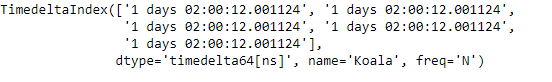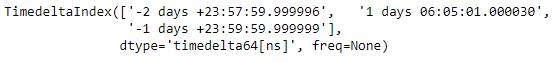Now we will append tidx2 at the end of tidx1.

 `# append tidx2 at the end of tidx1``tidx1.append(tidx2)`

Output :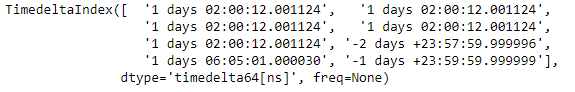As we can see in the output, the `TimedeltaIndex.append()` function has appended tidx2 at the end of tidx1.

Example #2: Use `TimedeltaIndex.append()` function to append a list of TimedeltaIndex object at the end of the given object.

 `# importing pandas as pd``import` `pandas as pd`` ` `# Create the first TimedeltaIndex object``tidx1 ``=` `pd.TimedeltaIndex(start ``=``'1 days 02:00:12.001124'``, periods ``=` `5``,``                                              ``freq ``=``'N'``, name ``=``'Koala'``)`` ` `# Create the second TimedeltaIndex object``tidx2 ``=` `pd.TimedeltaIndex(data ``=``[``'-1 days 2 min 3us 10ns'``,``                                 ``'1 days 06:05:01.000030'``,``                                 ``'-1 days + 23:59:59.999999'``])`` ` `# Create the third TimedeltaIndex object``tidx3 ``=` `pd.TimedeltaIndex(data ``=``[``'-3 days 02:10:00'``,``                                 ``'1 days 06:05:01.000030'``,``                                 ``'1 days 02:00:00'``], name ``=``'MyObjejct'``)`` ` `# Print the first TimedeltaIndex object``print``(tidx1)`` ` `# Print the second TimedeltaIndex object``print``(tidx2)`` ` `# Print the third TimedeltaIndex object``print``(tidx3)`

Output :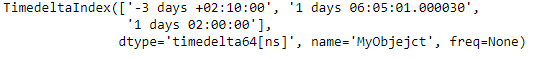Now we will append tidx2 and tidx3 at the end of tidx1.

 `# append tidx2 and tidx3 at the end of tidx1``tidx1.append([tidx2, tidx3])`

Output :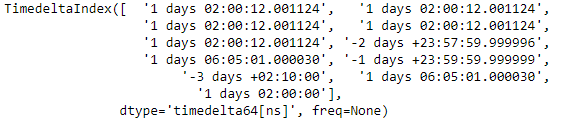As we can see in the output, the `TimedeltaIndex.append()` function has appended tidx2 and tidx3 at the end of tidx1.